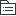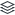# 生物必修一

734
|
215

## 相关模板推荐

•## 《分子与细胞》 —— 作品大纲

•走进细胞
• 细胞是生命体结构和功能的基本单位
• 生命系统的结构层次
• 细胞
• 组织
• 器官
• 系统
• 个体
• 种群
• 群落
• 生态系统
• 生物圈
• 使用显微镜观察细胞
• 原核细胞与真核细胞（生物）
• 有无细胞膜包被的细胞核
• 细胞学说及建立过程（施莱登·施旺）
• 动植物都以细胞为基本单位
•组成细胞的分子
• 元素
• C、H、O、N、P、S、K、Ca、Mg（大量元素）
• Fe、Mo、Zn、Cu、B（微量元素）
• 化合物
• 无机物
• 无机盐
• 有机物
• 蛋白质
• 核酸
• 糖类
• 脂质
• 蛋白质
• 组成单位
• 氨基酸（结构及种类）
• 结构及多样性
• 氨基酸数目
• 排列顺序
• 肽链空间结构
• 功能
• 结构蛋白
• 催化作用
• 运输作用
• 调节作用
• 免疫作用
• 核酸
• 分布
• 实验
• 甲基绿+DNA
• 变绿
• 吡罗红+RNA
• 变红
• 结论
• DNA细胞核线粒体
• 叶绿体RNA细胞质
• 组成
• DNA两条脱氧核苷酸链
• RNA一条核糖核苷酸
• 糖类
• 单糖
• 二糖
• 多糖
• 脂质
• 脂肪
• 磷脂
• 固醇
• 检测
• 蛋白质+双缩脲（紫）
• 还原糖+菲林（砖红色）
• 脂肪+苏丹Ⅲ/Ⅳ(橘黄/红）
• 淀粉+碘（蓝）
•细胞的基本结构
• 细胞膜
• 制备
• 哺乳动物红细胞
• 成分
• 脂质（磷脂）
• 蛋白质
• 糖类（少量）
• 功能
• 分隔
• 控制物质
• 信息交流
• 细胞质
• 基质
• 水、无机盐、脂质、糖类、氨基酸、核苷酸、多种酶
• 细胞器
• 单层膜
• 内质网、高尔基体、细胞器、液泡、溶酶体
• 双层膜
• 线粒体、叶绿体
• 无膜
• 核糖体、中心体（动物与低等植物）
• 协调配合
• 分泌蛋白
• 核糖体→内质网→高尔基体→细胞膜→胞外
• 生物膜系统
• 细胞器膜、核膜、细胞膜等
• 细胞核
• 功能
• 控制着细胞的代谢和遗传、遗传信息库
• 结构
• 核膜
• 双层膜，把核内物质和细胞质分开
• 染色质
• DNA+蛋白质，DNA是遗传信息的载体
• 核仁
• 与某种RNA的合成以及核糖体的形成有关
• 核孔
• 核质之间物质交换和信息交流
•细胞的物质输入和输出
• 物质跨膜实例
• 哺乳动物成熟红细胞（破裂）
• 植物成熟细胞（质壁分离）
• 细胞膜和其他生物膜都是选择透过性膜
• 流动镶嵌模式
• 探索历程
• 内容
• 磷脂双分子层
• 流动性
• 蛋白质
• 运输方式
• 主动运输
• 被动运输
• 自由扩散
• 无需载体蛋白、能量
• 协助扩散
• 载体蛋白、无需能量
• 胞吞胞吐
•细胞的能量供应和利用
• 作用
• 降低活化能，催化
• 本质
• 蛋白质，少量RNA
• 特性
• 高效性、专一性、作用条件温和
• ATP
• 结构
• A-P～P～P
• ATP的利用
• 细胞呼吸
• 有氧呼吸
• 无氧呼吸
• 光合作用
• 色素
• 叶绿素a、叶绿素b、叶黄素、胡萝卜素
• 叶绿体结构
• 外膜、内膜、基质、基粒
• 原理(探究历程)
• 过程
• 光反应
• 暗反应
• 化能合成作用
• 无机物氧化时释放的能量制造有机物
•细胞的生命历程
• 细胞增殖
• 有丝分裂
• 无丝分裂
• 减数分裂
• 细胞的分化
• 定义：形态、结构、生理功能
• 细胞全能性、干细胞
• 衰老和凋亡
• 衰老细胞特征
• 水、酶、色素、呼吸速率、膜通透性
• 细胞的凋亡
• 基因决定
• 自动结束
• 细胞癌变
• 定义
• 遗传物质
• 致癌因子
• 恶性增殖
• 特征
• 无限增殖
• 形态结构变化
• 表面
• 致癌因子
• 物理、化学、病毒
• 基因
• 原癌基因、抑癌基因